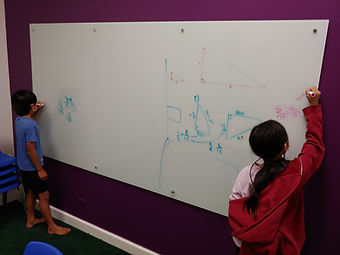## Ms. Mari

### Target 1​

###### Lesson Type:

Continuation

Geometry

:

Congruence

Identify similar triangles.

###### 1:

Prove that triangles are similar using SSS (Side-Side-Side), AA (Angle-Angle), and SAS (Side-Angle-Side).

###### 2:

Use the corresponding sides of similar triangles to determine the unknown length of a side.

###### 3:

Find the ratio of corresponding sides and use that ratio to find unknown lengths.

6th

###### Vocabulary:

SIMILAR TRIANGLES, SIDE-SIDE-SIDE THEOREM, ANGLE-ANGLE THEOREM, SIDE-ANGLE-SIDE THEOREM, CORRESPONDING SIDES AND ANGLES

Activities:

- Sorting Activity (Part 1) - Classified given similar triangles according to the similarity theorem shown (SSS, AA, SAS)

- Used proportions to solve for missing side lengths in similar triangles.### Home Exploration

###### Guiding Questions:## Absent Students:

### Target 2

:

###### 1:

Define similar figures as figures that have corresponding angle congruence and corresponding side proportionality.

###### 2:

Use the symbol “∼” to symbolize similarity.

6th

###### Vocabulary:

SIMILARITY, CORRESPONDING ANGLES

Activities:

- Sorting Activity (Part 2): After sorting the similar triangles according to the similarity theorems, students matched corresponding angles to construct similarity statements.### Home Exploration

###### Guiding Questions:### Target 3

:

###### Vocabulary:

Activities:### Home Exploration